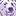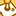# App Development

cancel
Showing results for
Did you mean:Contributor III

## Using a variable to format a measure by expression

Hello dear experts,

I want to make use of formatting a measure by expression, setting "Measure expression" in the KPI object.

I defined a variable in script to implement a desired formatting.

SET X = 'round(\$1/1000,0.1)&CHR(77)';

Data:
Dim, Sales
A, 150
A, 200
B, 240
B, 230
C, 410
C, 330
];

So far so good, it works with expression like \$(X(sum({<Dim={A}>}Sales))), but as soon as I need to use a comma in the set expression it does not work, like \$(X(sum({<Dim={A}, Dim>}Sales))).

I definitely want to make I use of the the option ""Measure expression" for formatting, my business case is more complex, but this examples shows that using a comma is problematic.

Does anybody have an idea how to solve this?

I also attached a sample QVF with this example.Labels (2)

• ### General Question

3 RepliesMVP

Hi, you can use a variable to store the expression and pass that variable:

``````SET expr1 = =Sum({<Dim={A}>} Sales);
SET expr2 = =Sum({<Dim={A},Dim>} Sales);
SET X = round(\$1/1000,0.1)&CHR(77);``````

\$(X(expr1))

\$(X(expr2))MVP & Luminary

I think there is no general and therefore satisfying solution possible. The comma will be always treated as parameter-delimiter for the variable. There is no possibility to change the behaviour or to mask the comma.

This means you will need workarounds to get such logics to work. This may be to replace the comma with another char by calling the variable and the variable itself contained also a logic to revert it again. This could become rather tedious ... maybe suitable for certain use-cases but rather not for general approaches.

Depending on the intended scenarios it's also thinkable to structure the variables different especially splitting them into several parts to avoid the comma-issue and other difficulties which could occur by nesting variables. Again not really recommended for a general use.

More practically but not without challenges is to avoid the commas by using different syntaxes and/or functions, for example:

... {< Dim1 = {'a'}, Dim2 = {'b'} >} ...

could be written as

... {< Dim1 = {'a'} > * < Dim2 = {'b'} >} ...

and

... {< Dim1 = {'a', 'b'} >} ...

could be written as

... {< Dim1 = {(a|b)} >} ...

- MarcusContributor

One intend would be to show numbers in a correctly rounded format with exactly one decimal and K M B ... afterwards for respective blocks of thousands.

currently we applied the following formatting varibale to almost all metrics in the apps:

SET vNumericalAbbreviation = 'dual(if(fabs(\$1)>=1000, if(fabs(\$1)>=1000000, round(\$1/1000000,0.1)&chr(77),round(\$1/1000,0.1)&chr(107)),round(\$1,0.1)),\$1)';

Moving the set expressions itself into script wouldn't be a viable option because then users can't do ad-hoc changes. Replacing the escaped character might work, though it will increase complexity even further.

Up until now I tried to separate the , parts e.g by using * but we recently stumbled across one expression which i didn't know how to rewrite. Splitting the whole expression into multiple blocks wouldn't work because the variable function needs to wrap the whole expression.

atm we use

sum({\$<Sonderfall,Kostenart_Zusammenfassung,Jahr={"\$(varCurrentYear)"}>*<Sonderfall,Kostenart_Zusammenfassung,timestamp={'\$(importZeitpunkt)'}>*<Sonderfall,Kostenart_Zusammenfassung,Risikokategorie-={3}>} [Risiko-Wert])

it is important that some dimensions are always filtered the same way while others should be filtered depening on the current analysis state. (dimensions not listed in the expression) In order to exclude "Sonderfall" and "Kostenart ..." dimensions from the filter we had to add them with , a simple | would not work because then there would also be entrys which should have been filtered by the other set modifiers... (unless you could use logical brackets in set expressions - which to my knowledge you can't